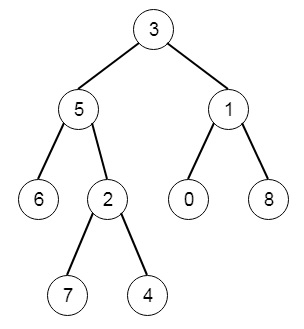# Lowest Common Ancestor of a Binary Tree in Python

PythonServer Side ProgrammingProgramming

Suppose we have a binary tree. we have to find the Lowest common ancestor nodes of two given nodes. The LCA of two nodes p and q is actually as the lowest node in tree that has both p and q as decedent. So if the binary tree is like [3,5,1,6,2,0,8,null,null,7,4]. The tree will be like −Here LCA of 5 and 1 is 3

To solve this, we will follow these steps −

• If the tree is empty, then return null
• if p and q both are same as root, then return root
• left := LCA of left subtree of the root using p and q
• right := LCA of right subtree of the root using p and q
• if left and right both are non-zero, then return root
• return left OR right

Let us see the following implementation to get better understanding −

## Example

Live Demo

class TreeNode:
def __init__(self, data, left = None, right = None):
self.data = data
self.left = left
self.right = right
def insert(temp,data):
que = []
que.append(temp)
while (len(que)):
temp = que
que.pop(0)
if (not temp.left):
if data is not None:
temp.left = TreeNode(data)
else:
temp.left = TreeNode(0)
break
else:
que.append(temp.left)
if (not temp.right):
if data is not None:
temp.right = TreeNode(data)
else:
temp.right = TreeNode(0)
break
else:
que.append(temp.right)
def make_tree(elements):
Tree = TreeNode(elements)
for element in elements[1:]:
insert(Tree, element)
return Tree
class Solution(object):
def lowestCommonAncestor(self, root, p, q):
if not root:
return None
if root.data == p or root.data ==q:
return root
left = self.lowestCommonAncestor(root.left, p, q)
right = self.lowestCommonAncestor(root.right, p, q)
if right and left:
return root
return right or left
ob1 = Solution()
tree = make_tree([3,5,1,6,2,0,8,None,None,7,4])
print(ob1.lowestCommonAncestor(tree, 5, 1).data)

## Input

[3,5,1,6,2,0,8,null,null,7,4]
5
1

## Output

3